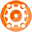Random

# Random

Description

Generates a random number or GUID.

Arguments

1.Random - Name of the Macro.

2.Type - The type of value to generate.  Options include Number (the default) for generating numbers and GUID for generating globally unique identifier values.

3.Minimum Value - The optional minimum value to use when generating a random value.  The default is 0.  Use only with the Number type.

4.Maximum Value - The optional maximum value to use when generating a random value.  The default is 100.  Use only with the Number type.

5.Format String - The optional .NET format string for formatting the output number.

Examples

<Random>

Returns a value between 1 and 100.  For example "67" is a potential output value.

<Random|GUID>

Returns a GUID value.  For example "B6C7195C-1B6D-4675-9F00-164E4519336B" is a potential output value.

<Random|Number|-5|5|000>

Returns a number between -5 and 5 and formats it with three significant digits.  For example "003" is a potential output value.

<Random|Number|1.0|10.0|0.00>

Returns a fractional number between 1 and 10 and formats it to include two digits behind the decimal point.  For example "8.57" is a potential output value.

Notes

When the minimum and maximum values are supplied the minimum must be less than the maximum value.  When either the minimum or maximum value are formatted to include a decimal point the resulting value will also have a decimal point.